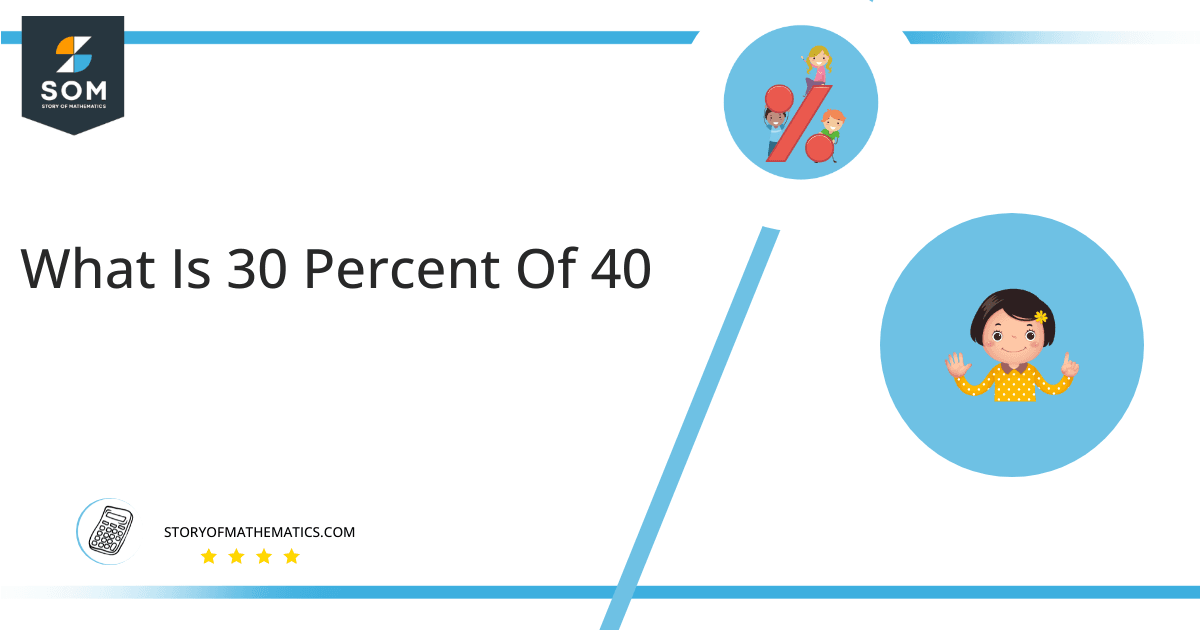# What Is 30 percent of 40 + Solution with Free Steps30 percent of forty yields the number twelve. This result is obtained by multiplying the factor 0.3 by 40.

This article describes the procedure for calculating a percentage of a specific number. We will concentrate on determining what 30 percent of 40 is.

## What Is 30 percent of 40?

The number 12 equals 30% of the number 40. Multiply the fraction 0.3 by the integer 40 to determine the solution.

The answer to the question is obtained by multiplying 40 by the fraction 30/100.

## How To Calculate 30 percent of 40?

30 percent of 40 can be determined using the following simple methods.### Step 1

30 percent of 40 expressed in mathematical form:

30 percent of 40 = 30% x 40

### Step 2

By Substituting the % symbol in 30% x 40 with the fraction 1/100:

30 percent of 40 = ( 30 x 1/100 ) x 40

### Step 3

Rearranging the above equation ( 30 x 1/100 ) x 40 gives:

30 percent of 40 = ( 30 x 40 ) / 100

### Step 4

Multiplying 30 with 40:

30 percent of 40 = ( 1200 ) / 100

### Step 5

Dividing 1200 by 100:

30 percent of 40 = 1200

Therefore, 30 percent of 40 is equivalent to 12.

With the help of the following pie chart, we can visualize what that 30 percent of 40 looks like.Figure 1: Pie Chart for 30 percent of 40

30 percent of forty is represented by the blue portion which equals 12. Overall, the area of the figure equates to the value of 40. The remaining 28, representing 70% of the total, are highlighted in purple.

The percentage is one of the most common methods for presenting data. Per hundred is the definition of percentage, and also the symbol for percentage is %. One percent (or 1%) is equivalent to one-hundredth of the total or whole; hence, it is computed by dividing the total or whole number by 100.

All the Mathematical drawings/images are created using GeoGebra.